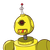# A e Euclid’s division algorithm to find the HCF of:135 and 225​

A e Euclid’s division algorithm to find the HCF of:
135 and 225

### 1 thought on “A e Euclid’s division algorithm to find the HCF of:<br />135 and 225<br />​”

1.45

Step-by-step explanation:

135 = 90 × 1 + 45

225 = 135 × 1 + 90Again, 45 ≠ 0, repeating the above step for 45, we get,

90 = 45 × 2 + 0

The remainder is now zero, so our method stops here. Since, in the last step, the divisor is 45, therefore, HCF (225,135) = HCF (135, 90) = HCF (90, 45) = 45.

Hence, the HCF of 225 and 135 is 45.